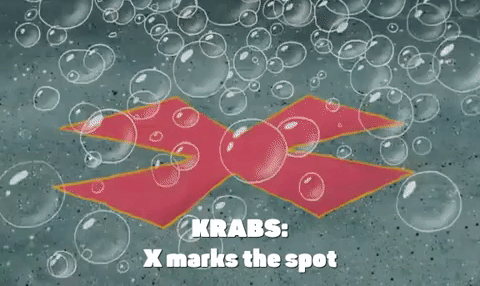IntroductionIntroduction# Proportions

INTRO

A ratio is a comparison of two quantities, and a proportion is an equation that lets us know two ratios are equal to each other.

So when we solve a proportion, we’re really just solving an equation, and we find that it’s easiest to do this when the ratios are written as fractions.

The top and bottom of each side of the equation form the 4 corners of an x, which means we can cross-multiply to solve for the unknown.

Remember, we can only cross-multiply when we have two ratios or fractions that are equal to each other - x marks the spot!We can also use cross-multiplication to double check that two ratios are equivalent and form a proportion:

 Cross-Multiply Result ✅ Equal / Proportion Not equal / Not a proportion
Check out our
Calculator
or explore our
Lesson
and
Practice

CALCULATOR

KEY STEPS

## How to Solve a Proportion

### Step 1. Write the ratios as fractions.

If the ratios are already in fraction format, continue to Step 2.

### Step 2. Cross-multiply if x marks the spot.

The top and bottom of each side of the equation form the 4 corners of an x.
This means we can multiply across the diagonals, and the products are equal.

### Step 3. Divide.

If solving for an unknown, divide both sides of the equation by the number it’s being multiplied by.
LESSON
Solving Proportions

## Solving Proportions

A proportion is an equation that lets us know two ratios are equal to each other.

When we write the ratios as fractions, we can use cross-multiplication to a proportion.

x marks the spot!

### Do we have to cross-multiply when the unknown variable is in the top?

While we can never go wrong with using cross-multiplication to solve proportions, if the unknown variable is in the top, we can just multiply both sides of the equation by its bottom.

Let’s walk through some example problems!
PRACTICE
Solving Proportions

## Practice: Solving Proportions

Question 1 of 10: Solve for if .

### Step 1. Write the ratios as fractions.

Since our ratios are already in fraction form, we can just continue to Step 2.

CONCLUSION
Incredible job, look at you go! Thanks for checking out this lesson ☺️🙏. Where to next?Leave Feedback